International
Tables for
Crystallography
Volume F
Crystallography of biological macromolecules
Edited by E. Arnold, D. M. Himmel and M. G. Rossmann

International Tables for Crystallography (2012). Vol. F, ch. 9.3, p. 235

## Section 9.3.2. Phase retrieval from single-particle diffraction data

D. Shapiroa*

aAdvanced Light Source, Lawrence Berkeley National Laboratory, 1 Cyclotron Rd, MS 2–400, Berkeley, CA 94720, USA
Correspondence e-mail: dashapiro@lbl.gov

### 9.3.2. Phase retrieval from single-particle diffraction data

| top | pdf |

The problem of phase retrieval is solved through successive application of constraints on the recovered object in the data and object spaces. Using the language of convex optimization, the mathematical operators which act on the data are projectors. The projector in the reciprocal (data) space forces the Fourier components to have the correct magnitude, while in object space finite support is enforced. To calculate the Fourier magnitude projector, one first needs to propagate the object density, ρ, to the data space by a Fourier transform, then replace the estimated magnitudes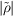with the measured ones, I1/2, and finally propagate back to real space. Using these transforms one simplifies the calculation of the projection, which becomes an element-wise operation on each recovered Fourier component. The forwardand inverse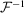transforms must be incorporated into the operator defined in real space Pm,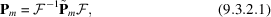where the measured Fourier magnitudes are enforced in Fourier space by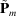. Using the Fourier basis, one simply replaces the estimated magnitudeswith the measured ones I1/2, [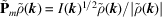]. Similarly, in the object space the finite support constraint is applied on a per pixel basis through multiplication by the support mask. The corresponding projector is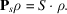Table 9.3.2.1lists the combination of projections used by the most popular algorithms.

 Table 9.3.2.1| top | pdf | Summary of various algorithms
 The algorithms are, from top to bottom: error reduction, solvent flipping, hybrid input–output, difference map, averaged successive reflections, hybrid projection–reflection and relaxed averaged alternating reflections. A reflection is defined by its associated projection as R = 2P − I, where I is the identity projection (Marchesini, 2007).
AlgorithmIteration ρ(n+1) =
ER PsPmρ(n)
SF RsPmρ(n)
HIO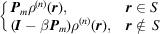DM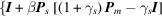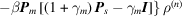ASR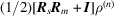HPR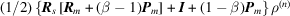RAAR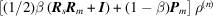The violation of the support constraint is used as an error metric to monitor the convergence towards the solution. The solution should have zero density outside the support mask, so the error can be defined as the total density outside the support area,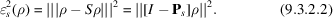Alternatively, the error metric can be defined in the data space as the difference between the measured and calculated magnitudes,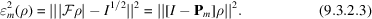In reality, the measured intensities are subject to noise which prohibits exact compliance with the constraints, so the error metrics cannot drop to zero. Although in most cases the algorithm can locate the global minimum, random noise will force fluctuations around the minimum. Once the algorithm reaches this steady-state regime, any particular iterate chosen as the solution would have a misleading degree of detail. On the other hand, the average of many fluctuating iterates would have reduced intensity in those Fourier components which are not reliably phased. The ratio of the average Fourier magnitude to the measured magnitude provides a measure of the stability, or reproducibility, of the retrieved phase information. This ratio as a function of spatial frequency is the phase retrieval transfer function (PRTF),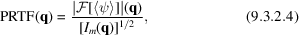where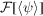is the Fourier transform of the final averaged image and Im is the measured intensity pattern. The PRTF is analogous to the differential phase residual of electron microscopy and, following Chapman et al. (2006), the resolution of a reconstruction is chosen as the spatial frequency at which the PRTF falls below a value of 0.5.

### References

Chapman, H. N., Barty, A., Marchesini, S., Noy, A., Hau-Riege, S. P., Cui, C., Howells, M. R., Rosen, R., He, H., Spence, J. C., Weierstall, U., Beetz, T., Jacobsen, C. & Shapiro, D. (2006). High-resolution ab initio three-dimensional X-ray diffraction microscopy. J. Opt. Soc. Am. A Opt. Image Sci. Vis. 23, 1179–1200.